GFG App
Open AppBrowser
Continue

# How to Create Anscombe’s Quartet in Excel?

Anscombe Quartet developed a situation that, despite the different datasets, with different scatter charts, data could have the same correlation values among them. Anscombe Quartet is famous to provide four data sets that tell us the importance of graphing and trend lines in the data. In this article, we will learn about Anscombe’s Quartet in excel.

## Anscombe’s Quartet

In 1973, Francis Anscombe told us the importance of graphing data before analyzing the data yet they have similar arithmetical identities. Anscombe’s Quartet is a group of four data sets that look identical with the same mean and correlation values but when creating a scatter chart they appear very differently. We are considering eleven data values in each data set.

## Anscombe’s Quartet in Excel

Step 1: Given the Anscombe Quartet dataset. The below 4 images, show the 4 datasets created by Anscombe.

DataSet 1: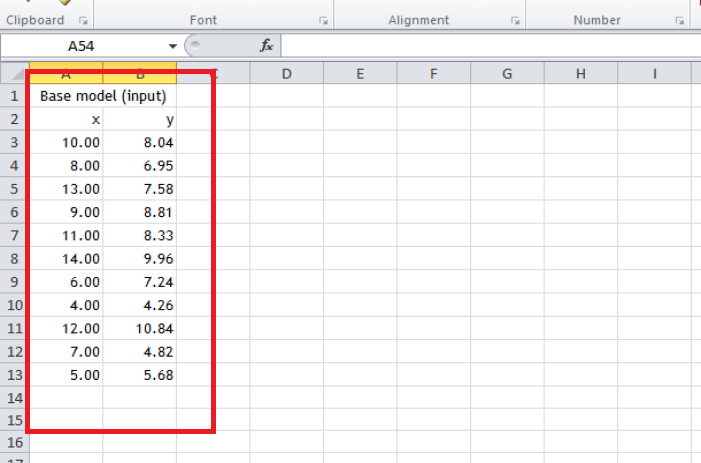DataSet 2: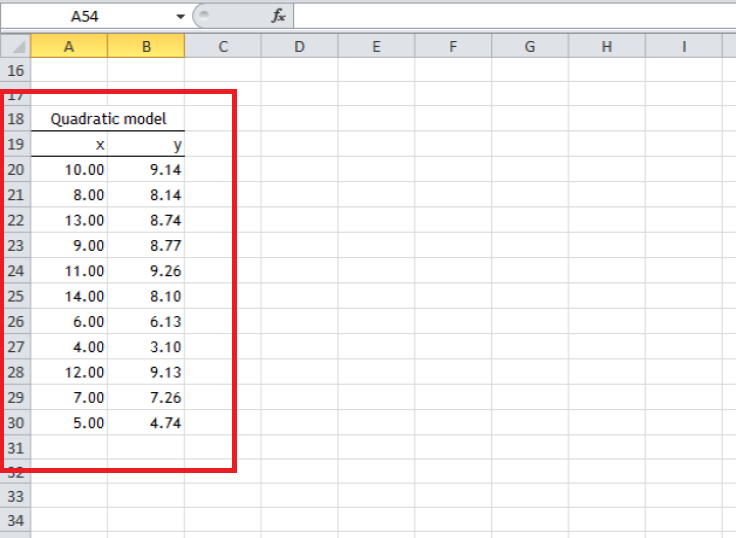DataSet 3: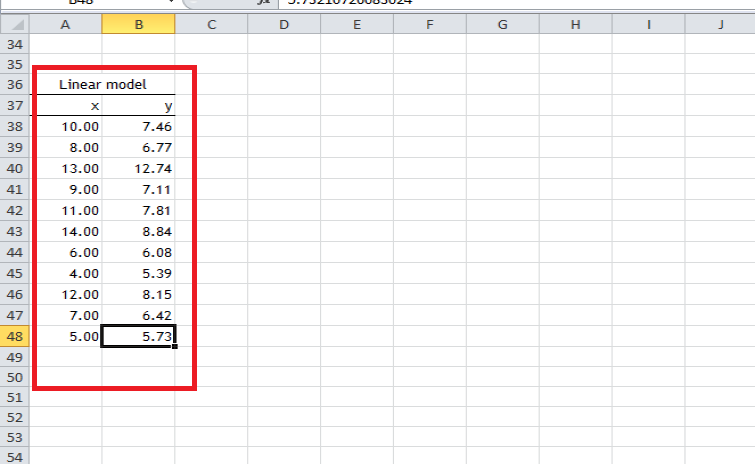Dataset 4: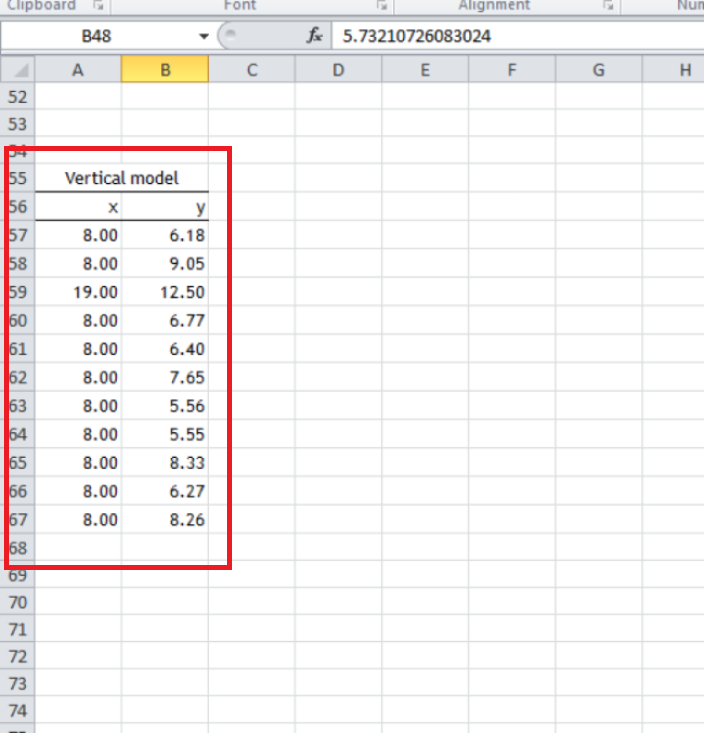Step 2: By physically viewing the data above. We are not able to infer what type of correlation data could have. To have better visual knowledge, we will create Scatter charts for each dataset. We will be showing the steps of creating a scatter chart, for dataset1, a similar process can be used to create charts for the rest of the datasets. Select the dataset1, A2:B13. Go to the Insert tab.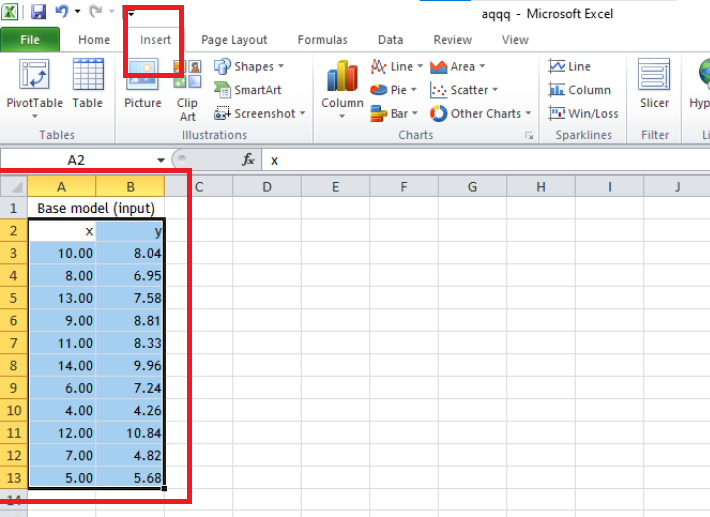Step 3: Under the Charts section, click on the Scatter option. Select the scatter chart from the list that appeared.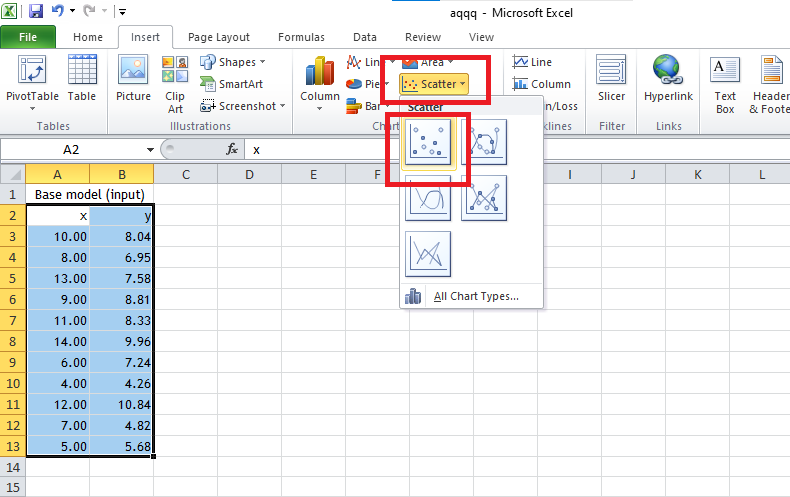Step 4: A scatter chart is created for dataset1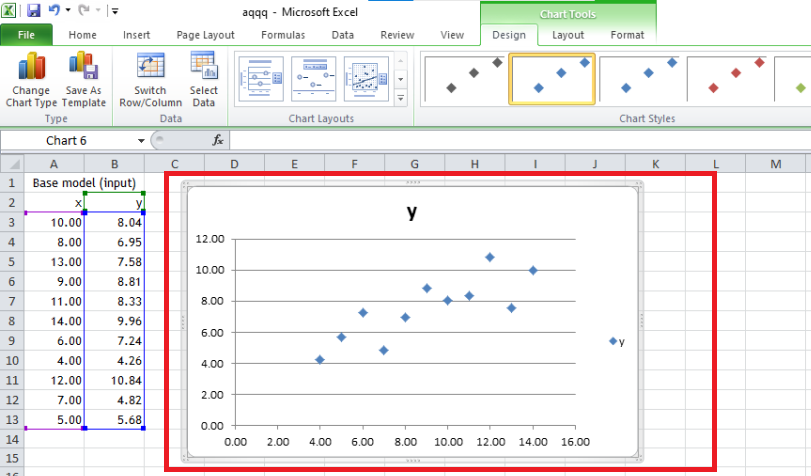Step 5: Repeat Steps 2,3, and 4, and create similar charts for dataset2, dataset3, and dataset4

Dataset 2: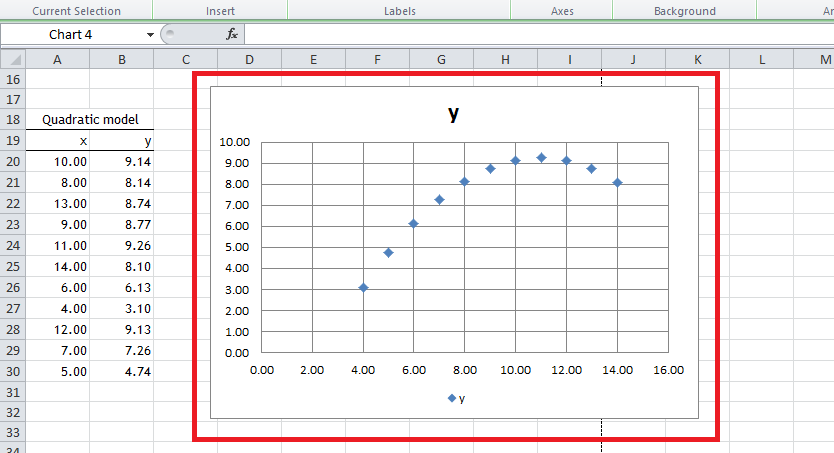Dataset 3: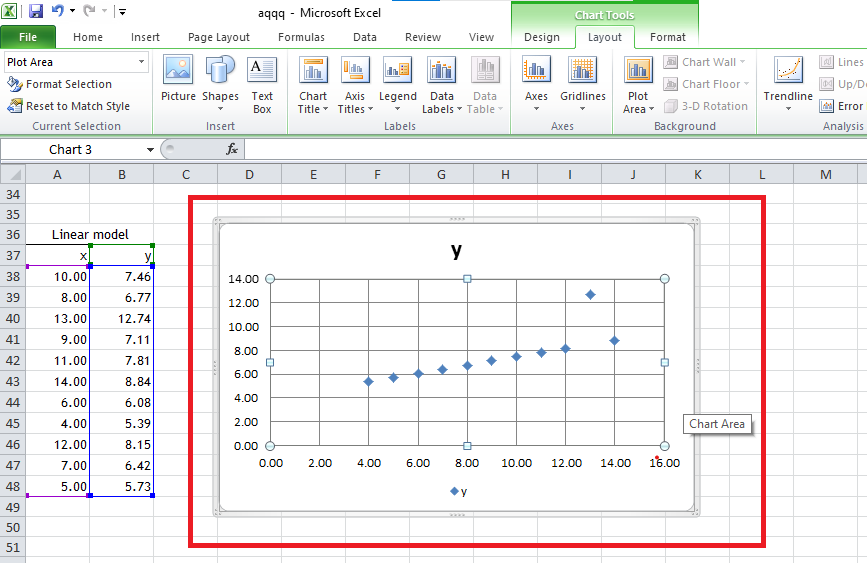Dataset 4: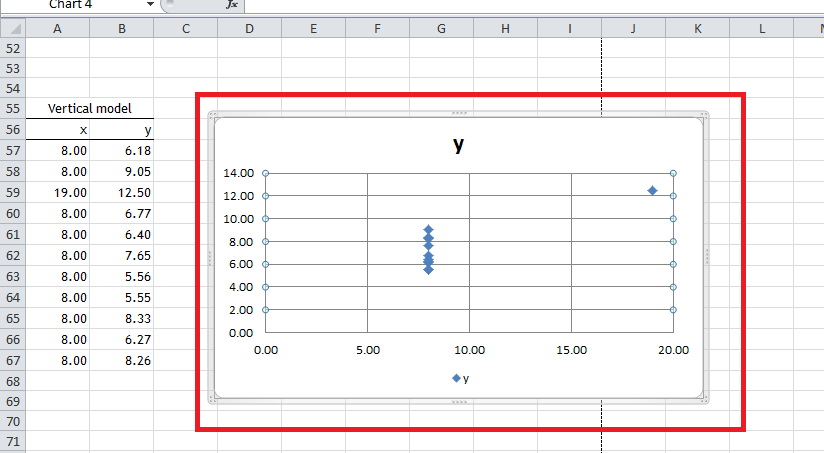Step 6: We can observe from the charts that, each chart has some different visualization in the scatter plot. Dataset1 has an evenly distribution, dataset2 has a parabolic shape, dataset3 is nearly a y = x line, and dataset 4 is nearly a straight line parallel to Y-Axis. Our next task is to add a trend line for each of the graphs, to have further insights into Anscombe’s quartet. Select the chart created for dataset1. Go to the Layout tab, and click on the Trendline option.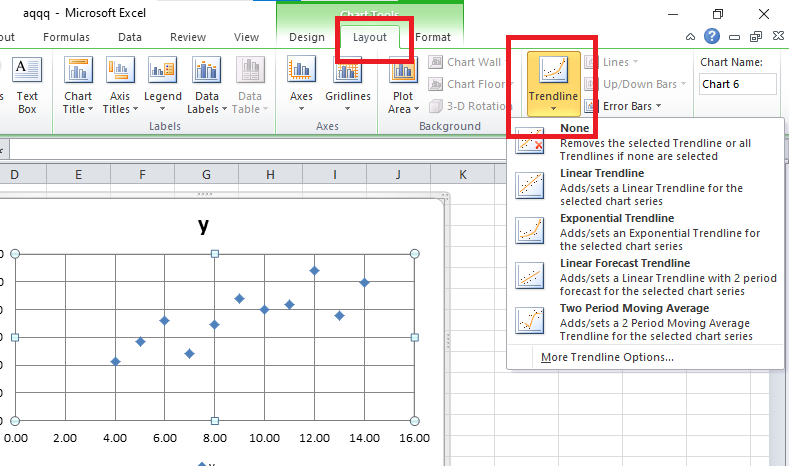Step 7: A drop-down list appears. Click on the Linear Trendline option.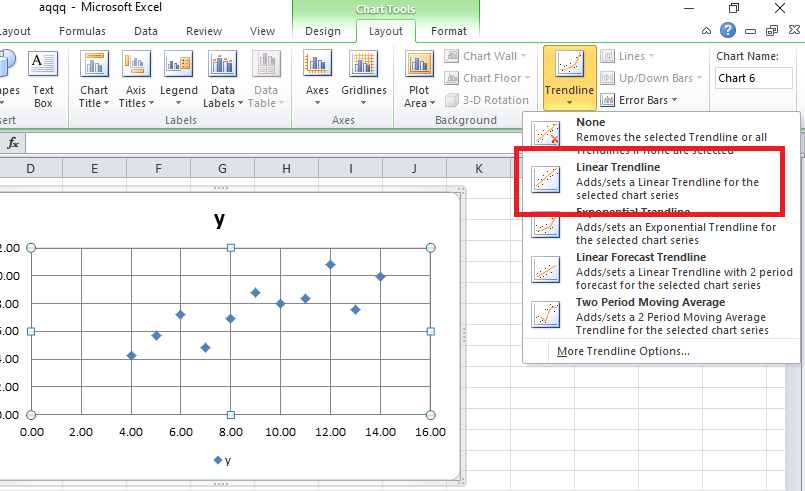Step 8: A trendline line appears in the graph.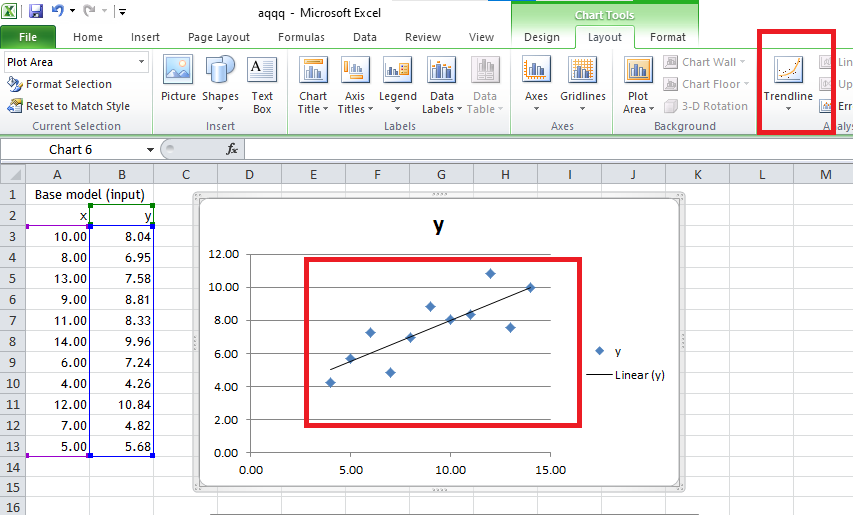Step 9: Repeat steps 6, 7, and 8, and add similar trendlines to dataset2, dataset3, and dataset4.

Dataset 2: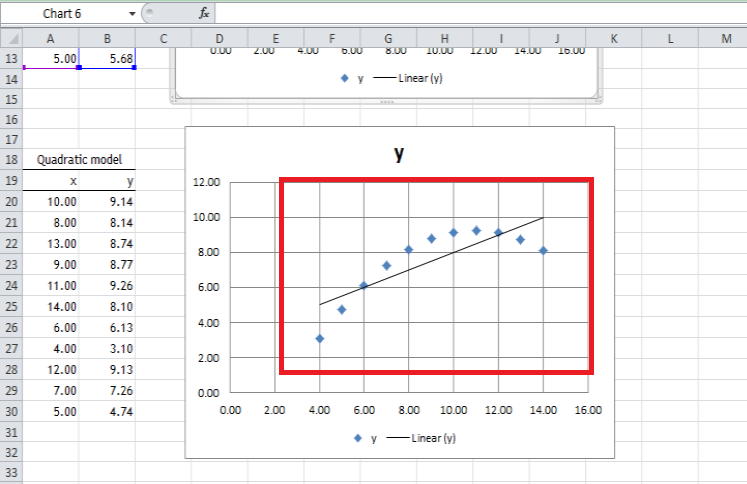Dataset 3: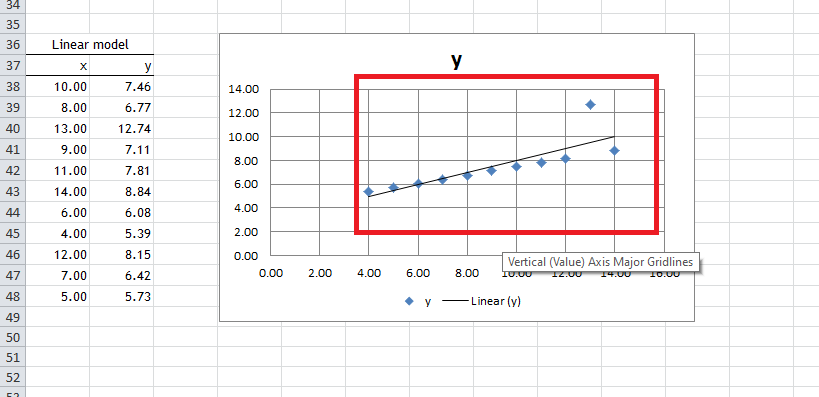Dataset 4: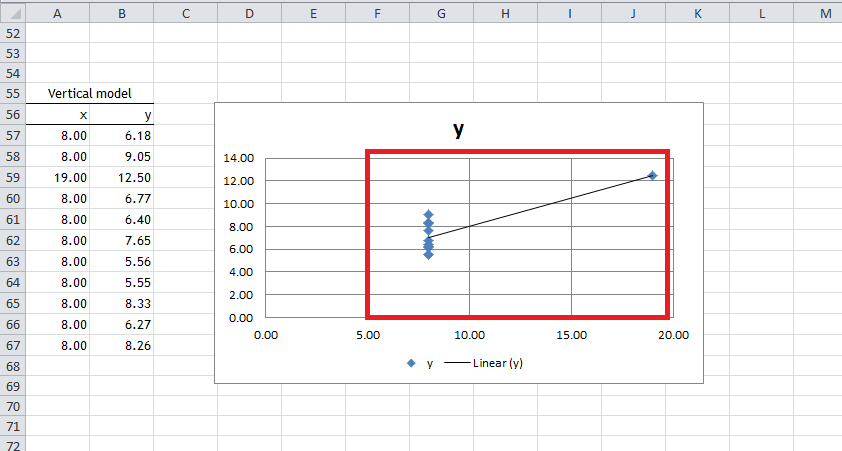Step 10: We can observe that the trend line looks similar for each of the datasets. Let us add the equation of the trend line to know more about the current datasets. Select the chart for dataset1. Go to the Layout tab, and click on Trendline.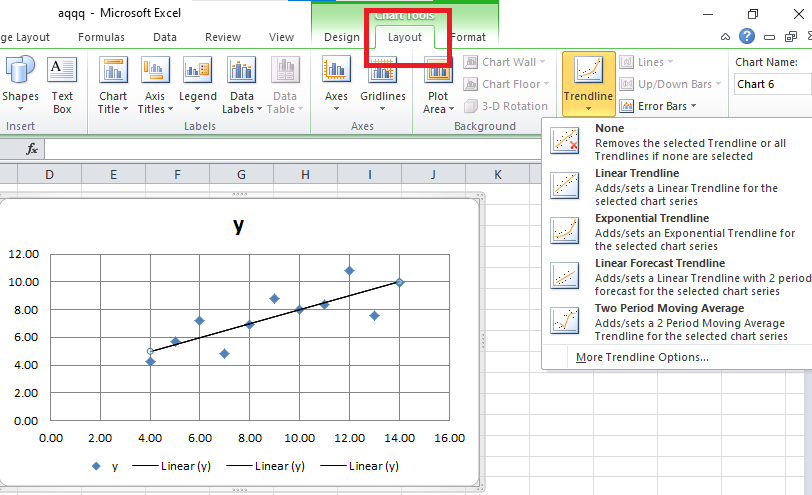Step 11: A drop-down list appears. Click on the More Trendline Options.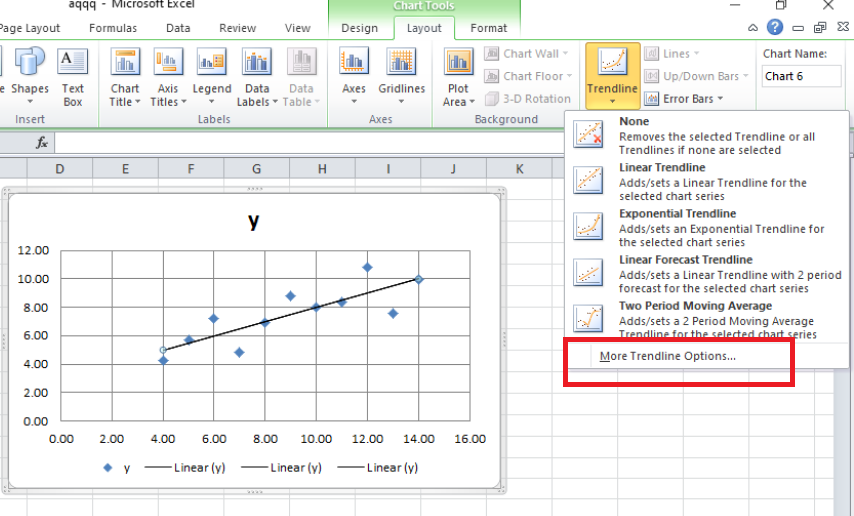Step 12: A new dialogue box, name, and Format Trendline appear on the screen. Check the box, Display equation on chart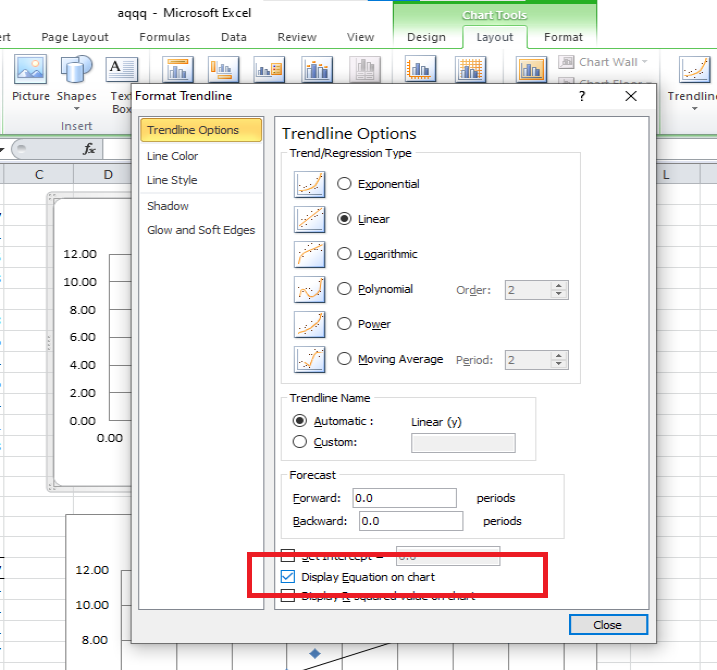Step 13: The trend line equation appears on the chart. The equation is y = 0.5x + 3 for dataset1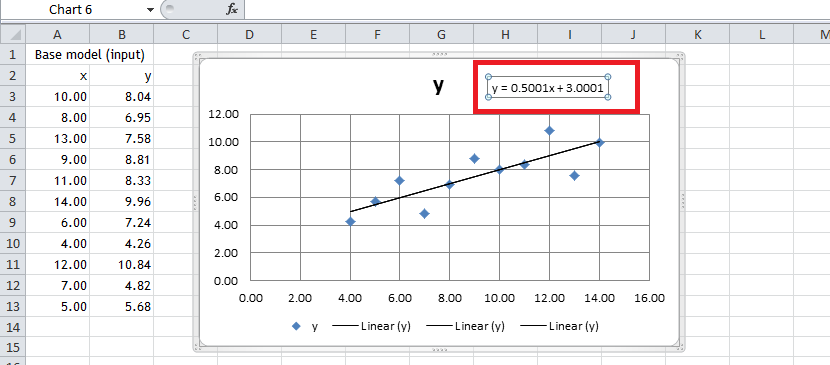Step 14: Repeat steps 10, 11, 12, and 13, and similarly add trendlines equation for dataset2, dataset3, and dataset4.

Dataset 2: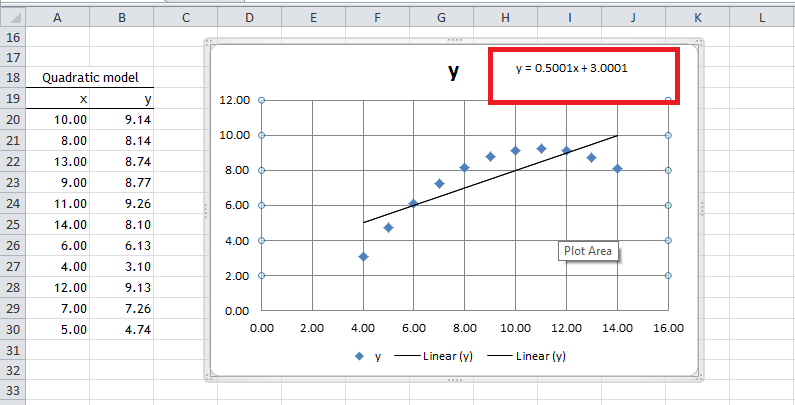Dataset 3: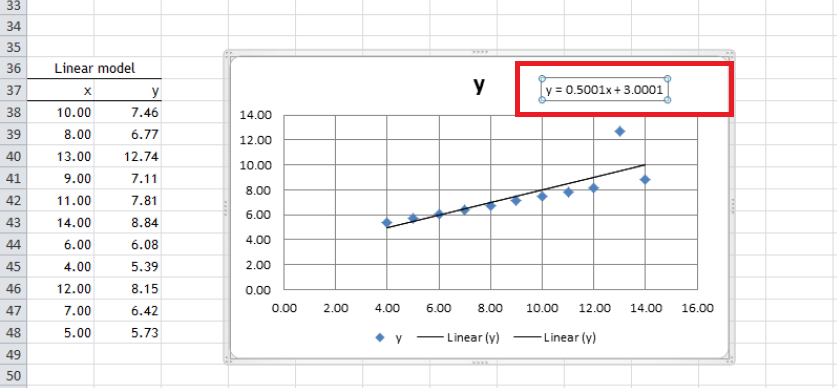Dataset 4: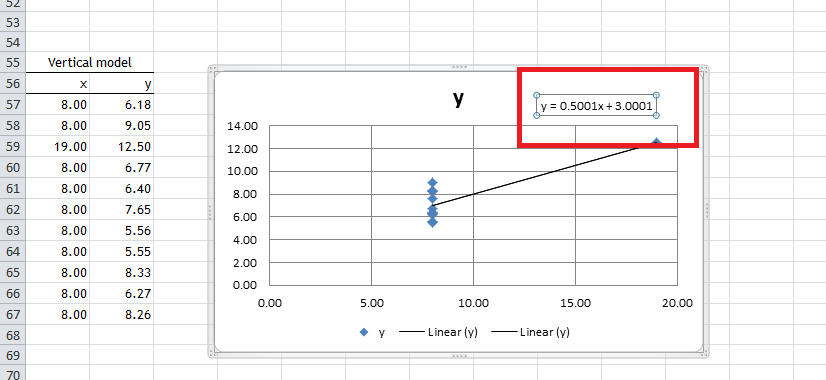We can observe that the equation of trendline is the same for all the datasets i.e. y = 0.5x + 3, despite the fact, that the scatter charts are very different from each other. Hence, the Anscombe quartet proved that only the summary statistics are not completely reliable, we need to look up the graphs also.

### Conclusion

By this, we conclude that graphical representation is important before analyzing the data whether they are identical or whether statistical identities are the same in nature. Graphical representations are different and have different relationships in all data sets.

My Personal Notes arrow_drop_up